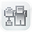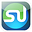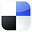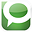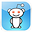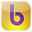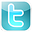What  Customers Are Saying  About  the Excel Statistical Master "We just started building statistical excel spreadsheets for our direct mail and online marketing campaigns, I purchased Excel Statistical Master to help fill in some of the blanks. Little did I know, this book has everything I could ever want to know about business statistics. Easy to follow and written so even a child could understand some of the most complex statistical theories. Thanks Mark!" Brandon Congleton Marketing Director www.worldprinting.com "After years of searching for a simplified statistics book, I found the Excel Statistical Master. Unlike the indecipherable jargon in the countless books I have wasted money on, the language in this book is plain and easy to understand. This is the best \$40 I have ever spent. " Mahdi Raghfar "I really like the Excel Statistical Master. It is incredibly useful. The explanations and videos in the manual are excellent. It has really made my work with statistics a LOT easier. I'm really glad that I came across the manual. If you're a student of business statistics, this e-manual is worth WAY more it's priced. I will use your manual as a reference for my MBA course this summer." Dr. Yan Qin Co-Director Nankai-Grossman Center for Health Economics and Medical Insurance
Excel
STATISTICAL
Master

Logistic Regression

Clear and Complete - WITH LOTS OF SOLVED PROBLEMS

# Logistic Regression

Video - How To Do Logistic Regression in Excel

Simple Explanation of How Logistic Regression Analysis Is Performed

Logistic regression is normally used to calculate the probability of an event occurring. Logistic regression analysis is performed by fitting data to a logit regression function logistic curve. The input variables (the predictor variables) can be numerical or categorical (dummy input variables).

Logistic regression is often called logit regression, the logistic model, or logit regression.

Using Logistic Regression

Logistic regression is used in social and medical sciences. For example, one medical use of logistic regression might be used to predict whether a person will have a stroke based upon the person's height, weight, and age. Marketers often use logistic regression to calculate the probability of whether or not a prospect will purchase.

Here is how the calculation is done (without wasting much time on theory):

The probability of the event occurring is given as follows:

P(X) = e**L/ (1+e**L)

The only variable in the above equation is L. L is called the Logit. The formula for L depends on the input variables. As a logistic regression example, if we were trying to predict the probability of a new prospect buying based upon the prospect's age and gender, then the equation for the Logit (L) would be the following:

L is the Logit and L = Constant + A*Age + B*Gender

We need to solve for Constant, A, and B. Once we have solved for these, we have solved for L. L can then be plugged into the probability equation P(X) above and we have the probability of the prospect purchasing.

So, the question is: How do we solve for Constant, A, and B?

We go back to our original customer and prospect data. We have recorded the age, gender, and whether each prospect purchased for all of our hundreds of previous prospects. For each of our previous prospect, we construct the following equation:

P(X)**Y * [ 1 - P(X) ]**(1-Y)

Y = 1 if the prospect purchased and Y = 0 if the prospect did not purchase.

P(X) is the probability equation and P(X) = e**L/ (1+e**L)

L is the Logit and L = Constant + A*Age + B*Gender

The equation P(X)**Y * [ 1 - P(X) ]**(1-Y) is maximized when P(X) approaches 1 (100%) when Y=1 and when P(X) approaches 0 when Y = 0.

Ultimately what we are doing is determining the Constant, A, and B that will maximize the sum of all P(X)**Y * [ 1 - P(X) ]**(1-Y) equations that we have calculated for each previous prospect.

This would be difficult to do by hand. It is best to use a tool like the Excel Solver. In fact you can consider Excel to be your logistic regression software. The attached video shows this being performed.

When you have found the ideal combination of (Constant, A, and B) that makes P(X) its most accurate for as many previous prospects as possible, the sum of all [ P(X)**Y * [ 1 - P(X) ]**(1-Y) ] equations will be maximized.

Once you have found that Constant, A, and B that maximizes that sum, you can then plug the Constant, A, and B into the Logit equation:

L = Constant + A*Age + B*Gender

After this, you have the correct Logit (L), which can then be plugged into the probability equation: P(X) = e**L/ (1+e**L) and you have the most accurate probability of whether your new prospect will purchase.

The attached video provides a logistic regression tutorial of how the calculation is performed using the Excel Solver. You can consider Excel to your logistic regression software.

If You Like This, Then Share It...